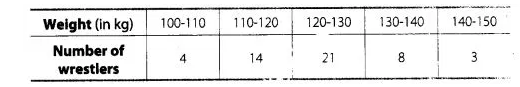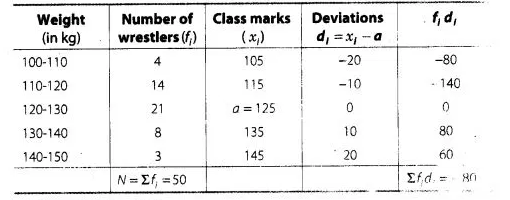# The weights (in kg) of 50 wrestlers are recorded in the following table.

Question:

The weights (in kg) of 50 wrestlers are recorded in the following table.

Find the mean weight of the wrestlers.Solution:

We first find the class mark of each class and then proceed as follows$\therefore$ Assumed mean $(a)=125$,

Class width $(h)=10$ and total observation $(N)=50$

By assumed mean method.

Mean $(\bar{x})=a+\frac{\Sigma f_{j} d_{i}}{\Sigma f_{i}}$

$=125+\frac{(-80)}{50}$

$=125-1.6-123.4 \mathrm{~kg}$